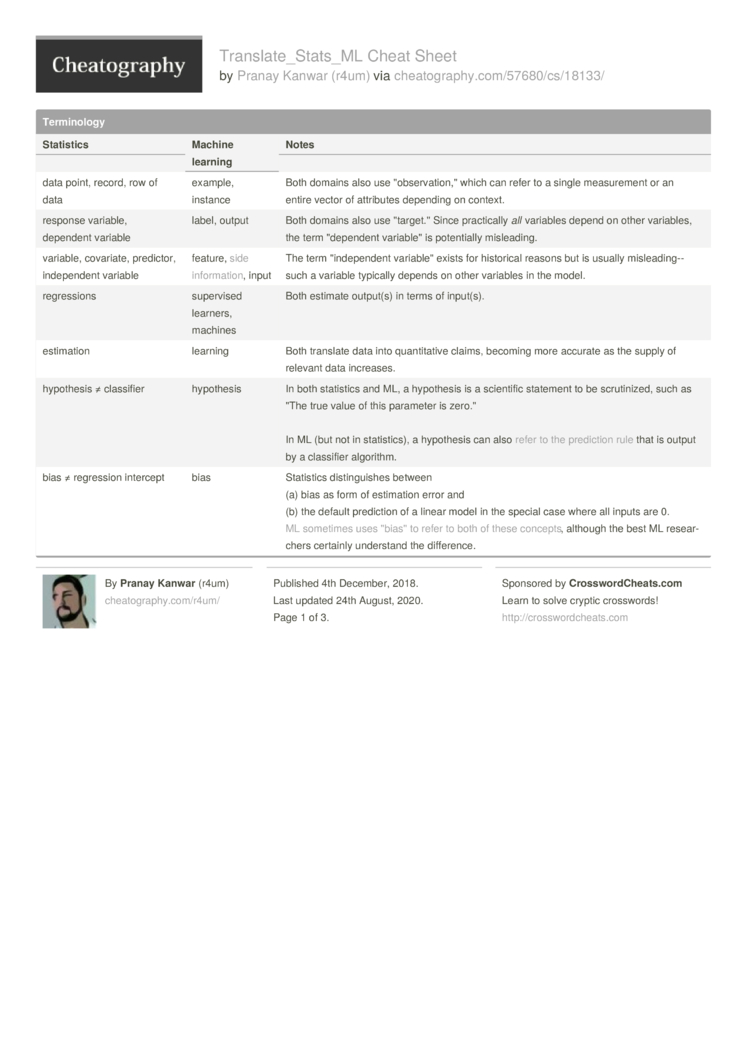# Translate_Stats_ML Cheat Sheet by r4um

Translating Between Statistics and Machine Learning

### Termin­ology

 Statistics Machine learning Notes data point, record, row of data example, instance Both domains also use "­obs­erv­ati­on,­" which can refer to a single measur­ement or an entire vector of attributes depending on context. response variable, dependent variable label, output Both domains also use "­tar­get." Since practi­cally all variables depend on other variables, the term "­dep­endent variab­le" is potent­ially mislea­ding. variable, covariate, predictor, indepe­ndent variable feature, side information, input The term "­ind­epe­ndent variab­le" exists for historical reasons but is usually mislea­din­g--such a variable typically depends on other variables in the model. regres­sions supervised learners, machines Both estimate output(s) in terms of input(s). estimation learning Both translate data into quanti­tative claims, becoming more accurate as the supply of relevant data increases. hypothesis ≠ classifier hypothesis In both statistics and ML, a hypothesis is a scientific statement to be scruti­nized, such as "The true value of this parameter is zero." In ML (but not in statis­tics), a hypothesis can also refer to the prediction rule that is output by a classifier algorithm. bias ≠ regression intercept bias Statistics distin­guishes between(a) bias as form of estimation error and(b) the default prediction of a linear model in the special case where all inputs are 0. ML sometimes uses "­bia­s" to refer to both of these concepts, although the best ML resear­chers certainly understand the differ­ence. Maximize the likelihood to estimate model parameters If your target distri­bution is discrete (such as in logistic regres­sion), minimize the entropy to derive the best parame­ters. If your target distri­bution is contin­uous, fine, just maximize the likeli­hood. For discrete distri­but­ions, maximizing the likelihood is equivalent to minimizing the entropy. Apply Occam's razor, or encode missing prior inform­ation with suitably uninfo­rmative priors The principle of maximum entropy is conceptual and does not refer to maximizing a concrete objective function. The principle is that models should be conser­vative in the sense that they be no more confident in the predic­tions than is thoroughly justified by the data. In practice this works out as deriving an estimation procedure in terms of a bare-m­inimum set of criteria as exempl­ified here or here. logist­ic/­mul­tin­omial regression maximum entropy, MaxEnt They are equivalent except in special multin­omial settings like ordinal logistic regres­sion. Note that maximum entropy here refers to the principle of maximum entropy, not the form of the objective function. Indeed, in MaxEnt, you minimize rather than maximize the entropy expres­sion. X causes Y if surgical (or randomized contro­lled) manipu­lations in X are correlated with changes in Y X causes Y if it doesn't obviously not cause Y. For example, X causes Y if X precedes Y in time (or is at least contemporaneous) The stats definition is more aligned with common­-sense intuition than the ML one proposed here. In fairness, not all ML practi­tioners are so abusive of causation termin­ology, and some of the blame belongs with even earlier abuses such as Granger causality. structural equations model Bayesian network These are nearly equivalent mathem­ati­cally, although interp­ret­ations differ by use case, as discussed. sequential experi­mental design active learning, reinfo­rcement learning, hyperp­ara­meter optimi­zation Although these four subfields are very different from each other in terms of their standard use cases, they all address problems of optimi­zation via a sequence of querie­s/e­xpe­rim­ents.3 Pages
//media.cheatography.com/storage/thumb/r4um_translate-stats-ml.750.jpg

PDF (recommended)